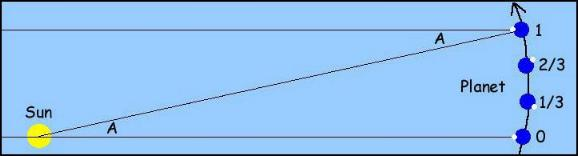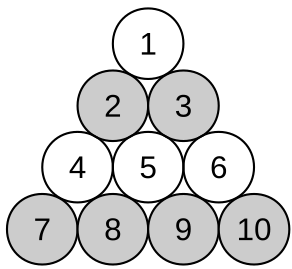________________

With the semester just about to end, Coach Newton is a week away from his retirement. He finishes up with a pair of geometry problems, his favorite kind.

________________Thirds Squared

Start with any square ABCD. Connect vertex A by a new line segment to a point one third of the way from B to C, vertex B to a point one third of the way from C to D, vertex C to a point one third of the way from D to A, and vertex D to a point one third of the way from A to B. These four added segments create four points of intersection in the interior of ABCD. The four intersection points are the vertices of a smaller square.

What is the ratio of the area of the smaller square to the area of ABCD?

3-4-5 Square

This time, suppose there is a square ABCD with a point E on edge BC and a point F on edge CD such that the area of triangle ABE is 3, of ADF is 4, and of EFC is 5.

What’s the area of the square?

Solutions to week 89

World Turns. On average, given that there is a leap year about every four years (ignoring the small corrections for leap years each century), to the nearest day there are 365 1/4 days per year. So you might think that means the earth has rotated 365 1/4 turns. However, since in the course of one day the Earth has revolved a bit around the Sun, after exactly one rotation, the Sun will not be in the same place in the sky. Since the direction of the Earth’s rotation is the same as the direction of Earth’s revolution around the Sun (both counter-clockwise if viewed from the North Star, a fact you just need to look up), the Earth has to rotate just slightly farther than one full rotation in order for the Sun to once again appear in the same position in the sky. All of those extra little bits of rotation add up to exactly one extra turn for the Earth, corresponding to the fact that the Earth makes one full trip around the Sun during the year. So on average, to the nearest quarter-turn, the Earth rotates 366 1/4 times per year. The diagram below from Prof. Courtney Seligman’s online astronomy text should help visualize the extra bit of rotation that the Earth makes each day.Water Slide.You can transform the first configuration to the second by moving three glasses, as follows: Slide the glass labeled 1 to nestle just below glasses 8 and 9 (into what will be position J in the final configuration). Pick up glass 7, pour it into glass 4, and set it down just touching glasses 2 and 4 (in what will be position A). Finally, pick up glass 10, pour it into glass 6, and set it down just touching glasses 3 and 6 (in what is now position D). The challenge cannot be performed by moving fewer than three glasses, because any way you might slide the final diagram over the first, you can make at most seven glasses coincide in location (the central hexagon of seven glasses in each diagram). So the remaining three glasses must move. And of course, if you don’t pour any water, it will take more than three moves, since you can’t get seven of the glasses to correspond both in position and full/empty status just by sliding the diagrams.

Recent Weeks

Links to all of the puzzles and solutions are on the Complete Varsity Math page.

Come back next week for answers and more puzzles.

[asciimathsf]